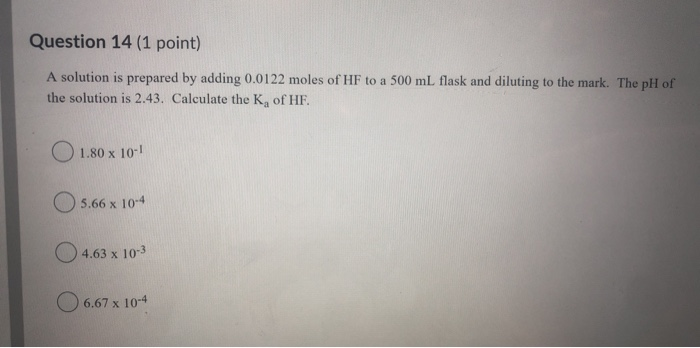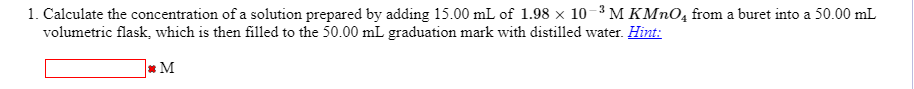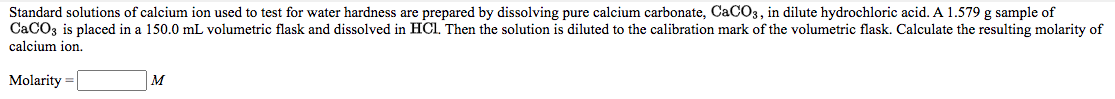Question

# Given: Ka(HF) = 6.3EE-04 A solution is prepared by adding 0.057 moles of calcium fluoride to...

Given: Ka(HF) = 6.3EE-04 A solution is prepared by adding 0.057 moles of calcium fluoride to a 150 mL volumetric flask and diluting to the calibration mark. What is the pH of this solution?

Number of moles of CaF2(aq) = 0.057 mol

Volume of solution = 150 ml = 150 L / 1000 = 0.150 L

[CaF2] = 0.057 mol / 0.150 L = 0.38 mol/L = 0.38 M

In aqueous solution, [F^-(aq)] = 2 * [CaF2(aq)] = 2 * 0.38 M = 0.76 M

We know . Kb = Kw / Ka = 1.00 * 10^-14 / 6.3 * 10^-4 = 0.1587 * 10^-10

Consider a hydrolysis reaction of F^-(aq)

F^-(aq) + H2O (l) ----> OH^-(aq) + HF(aq)

Initial Conc. 0.76 0 0

Change in Conc. - x. +x +x

Final Conc. 0.76 - x . x. x

Kb = [OH^-][HF] / [F^-] = (x)(x) / (0.76 - x) = x^2 / 0.76

Since Kb is very small hence 0.76 - x ~ 0.76

x^2 = 0.76 * Kb = 0.76 * 0.1587 * 10^-10 = 0.1206 * 10^-10

x = √(0.1206 * 10^-10) = 0.3473 * 10^-5 = 3.473 * 10^-6

Since [OH^-] = x M hence [OH^-] = 3.473 * 10^-6 M

We know

pOH = - log[OH^-] = - log (3.473 * 10^-6) = - log 3.473 + 6 log 10 = - 0.54 + 6 * 1 = 5.46

We know pH = 14.00 - pOH = 14.00 - 5.46 = 8.54

pH = 8.54

#### Earn Coins

Coins can be redeemed for fabulous gifts.

Similar Homework Help Questions
• ### Question 14 (1 point) A solution is prepared by adding 0.0122 moles of HF to a...Question 14 (1 point) A solution is prepared by adding 0.0122 moles of HF to a 500 ml flask and diluting to the mark. The pH of the solution is 2.43. Calculate the Ky of HF. O 1.80 x 10-1 5.66 x 10-4 4.63 x 10-2 6.67 x 10-4

• ### What is the pH of a solution made by dissolving 5.96 grams of calcium fluoride in...

What is the pH of a solution made by dissolving 5.96 grams of calcium fluoride in enough water to make 610 mL of solution? The Ka for HF is 6.8x10–4.

• ### What is the pH of a solution made by dissolving 2.66 grams of calcium fluoride in...

What is the pH of a solution made by dissolving 2.66 grams of calcium fluoride in enough water to make 450 mL of solution? The Ka for HF is 6.8x10–4.

• ### What is the pH of a solution made by dissolving 4.31 grams of calcium fluoride in...

What is the pH of a solution made by dissolving 4.31 grams of calcium fluoride in enough water to make 640 mL of solution? The Ka for HF is 6.8x10–4.

• ### What is the pH of a solution made by dissolving 5.06 grams of calcium fluoride in...

What is the pH of a solution made by dissolving 5.06 grams of calcium fluoride in enough water to make 7.0×102 mL of solution? The Ka for HF is 6.8x10–4.

• ### Standard solutions of calcium ion used to test for water hardness are prepared by dissolving pure...

Standard solutions of calcium ion used to test for water hardness are prepared by dissolving pure calcium carbonate, CaCO3, in dilute hydrochloric acid. A 1.212 g sample of CaCO3 is placed in a 200.0 mL volumetric flask and dissolved in HCI. Then the solution is diluted to the calibration mark of the volumetric flask. Calculate the resulting molarity of calcium ion.

• ### A solution is prepared by adding 0.5630 g of powdered milk into a 2.5E+02 mL volumetric flask and adding 30 mL of 1.0 M...

A solution is prepared by adding 0.5630 g of powdered milk into a 2.5E+02 mL volumetric flask and adding 30 mL of 1.0 M KCL and diluting to volume with DI water. The concentration of a milk sample is determined to be 6.420×10-4 M Ca2+ based on linear regression analysis of calcium standard solutions. What is the concentration of Ca2+ in the milk sample (wt/wt %)? [MW Ca = 40.078 g/mole]

• ### Calculate the concentration of a solution prepared by adding 15.00 mL of 1.98 × 10 −...Calculate the concentration of a solution prepared by adding 15.00 mL of 1.98 × 10 − 3 M K M n O 4 from a buret into a 50.00 mL volumetric flask, which is then filled to the 50.00 mL graduation mark with distilled water 1. Calculate the concentration of a solution prepared by adding 15.00 mL of 1.98 x 10-3M KMnO, from a buret into a 50.00 mL volumetric flask, which is then filled to the 50.00 mL graduation...

• ### A stock solution of Cu2+(aq) was prepared by placing 0.9157 g of solid Cu(NO3)2∙2.5 H2O in...

A stock solution of Cu2+(aq) was prepared by placing 0.9157 g of solid Cu(NO3)2∙2.5 H2O in a 100.0-mL volumetric flask and diluting to the mark with water. A standard solution was then prepared by transferring 2.00 mL of the stock solution to a second 25.00-mL volumetric flask and diluting to the mark. What is the concentration (in M) of Cu2+(aq)in the stock solution? What is the concentration (in M) of Cu2+(aq)in the standard solution?

• ### Standard solutions of calcium ion used to test for water hardness are prepared by dissolving pure...Standard solutions of calcium ion used to test for water hardness are prepared by dissolving pure calcium carbonate, CaCO3, in dilute hydrochloric acid. A 1.579 g sample of CaCO3 is placed in a 150.0 mL volumetric flask and dissolved in HCl. Then the solution is diluted to the calibration mark of the volumetric flask. Calculate the resulting molarity of calcium ion. Molarity M For convenience, one form of sodium hydroxide that is sold commercially is the saturated solution. This solution...